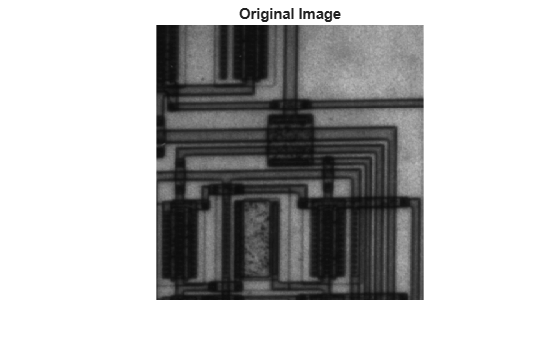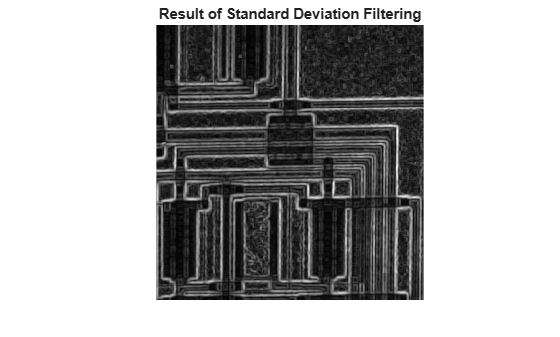# stdfilt

Local standard deviation of image

## Syntax

``J = stdfilt(I)``
``J = stdfilt(I,nhood)``

## Description

example

````J = stdfilt(I)` performs standard deviation filtering of image `I` and returns the filtered image `J`. The value of each output pixel is the standard deviation of the 3-by-3 neighborhood around the corresponding input pixel. For pixels on the borders of `I`, `stdfilt` uses symmetric padding. In symmetric padding, the values of padding pixels are a mirror reflection of the border pixels in `I`.```
````J = stdfilt(I,nhood)` specifies the neighborhood, `nhood`, used to compute the standard deviation.```

## Examples

collapse all

This example shows how to perform standard deviation filtering using `stdfilt`. Brighter pixels in the filtered image correspond to neighborhoods in the original image with larger standard deviations.

Read an image into the workspace.

`I = imread('circuit.tif');`

Perform standard deviation filtering using `stdfilt`.

`J = stdfilt(I);`

Show the original image and the processed image.

```imshow(I) title('Original Image')``````figure imshow(J,[]) title('Result of Standard Deviation Filtering')```## Input Arguments

collapse all

Image to be filtered, specified as a numeric array or logical array of any dimension.

Data Types: `single` | `double` | `int8` | `int16` | `int32` | `int64` | `uint8` | `uint16` | `uint32` | `uint64` | `logical`

Neighborhood, specified as a numeric or logical array containing `0`s and `1`s. The size of `nhood` must be odd in each dimension.

By default, `stdfilt` uses the neighborhood `true(3)`. `stdfilt` determines the center element of the neighborhood by ```floor((size(nhood) + 1)/2)```.

To specify neighborhoods of various shapes, such as a disk, use the `strel` function to create a structuring element object of the desired shape. Then extract the neighborhood from the `neighborhood` property of the structuring element.

Data Types: `single` | `double` | `int8` | `int16` | `int32` | `int64` | `uint8` | `uint16` | `uint32` | `uint64` | `logical`

## Output Arguments

collapse all

Filtered image, returned as a numeric array of the same size as the input image `I`. The data type of `J` is `double`.

## Tips

• The `double` array `J` contains standard deviation values, which can exceed the range [0, 1]. Because some Image Processing Toolbox™ functions expect inputs of type `double` to be in the range [0, 1], to pass `J` as an input argument to these functions, use the `rescale` function to rescale the values of `J` to [0, 1].

• If the image contains `Inf`s or `NaN`s, then the behavior of `stdfilt` is undefined. Propagation of `Inf`s or `NaN`s might not be localized to the neighborhood around the `Inf` or `NaN` pixel.

## Version History

Introduced before R2006a